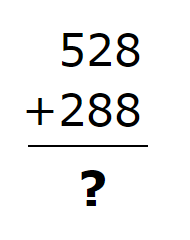Friday, September 17, 2021# Vertical addition (m3.nbt.2) math practice

-

This test question, released by the Partnership for the Assessment of Readiness for College and Career (PARCC), is discussed here in the interest of helping third-grade students, their parents, and friends understand more clearly the knowledge and skills that third graders should have in math by the end of the school year (2018 #2).For this problem, you have to type a number into a box that represents the sum of 528 and 288. The correct answer is 816.

• If your answer is in the 700s, you forgot to “carry” the number “1” from the tens place.
• If your answer has a “0” in the tens place, you forgot to carry the “1” from the ones place.

You should always start addition problems like this from the ones place, which for whole numbers is the number on the right. The ones place of the top number is 8, and the ones place of the bottom number is also 8. Adding these gives 8 + 8, which equals 16. As you know from place value, 16 is the same as 6 plus one 10. What that means in the addition problem is that underneath the ones place in the problem write down the “6” from your answer, which is “6,” and put the one 10 in the the tens place by writing a “1” there.

Next, add the three numbers in the tens column: the 2 from the top number, the 8 from the bottom number, and the 1 that you carried over from the ones column. 2 tens + 8 tens + 1 ten = 11 tens. 11 tens is the same as 1 ten plus 1 hundred, so you now write down the 1 ten in the tens place of your answer and carry the one hundred to the hundreds place.

Finally, add the three numbers in the hundreds column: 5 hundreds + 2 hundreds + 1 hundred equals 8 hundreds. When you write the “8” in the hundreds place of the problem, you see that the resulting answer is 816.

If You Don't Understand This Problem
When we write the number “528,” what does that mean. There’s a “5” in the hundreds place, so that’s 500. There’s a “2” in the tens place, so that’s 20. And there’s an “8” in the ones place, just that’s just 8. We can write this about the number:$528 = 500 + 20 + 8$

Likewise, with the second number, it can be broken down by place value as well.$288 = 200 + 80 + 8$

We can then add the two numbers piece by piece. 8 + 8 in the ones place is 16, or 6 plus one 10. Then we add the tens place: 80 + 20 + the 10 from the 16 in the ones column, and that makes 110, which is actually 11 tens, or 1 ten plus 1 hundred. For now, “carry” the 1 hundred to the hundreds place and add it up there.

That makes 500 + 200 + 100 in the hundreds place, which is 800. Our final answer is:$800 + 10 + 6 = 816$

You can learn more with this type of question by practicing different “strategies” for performing the three-digit addition. Some of the different strategies include:

• the traditional way, right to left
• an approach using just place value
• explaining that the answer is correct by subtracting one of the numbers from the sum

It would be best if students could explain what they’re doing (thinking out loud) as they solve the problems using each of these strategies.

Internet Resources

## Information for Teachers

This problem tests students’ understanding of the Common Core mathematics standard 3.NBT.A.2, which says that by the end of third grade, you should have the ability to “fluently add and subtract within 1000 using strategies and algorithms based on place value, properties of operations, and/or the relationship between addition and subtraction.”

Note that “fluency” means much more than being able to add and subtract quickly. The National Council of Teachers of Mathematics defines procedural fluency as “the ability to apply procedures accurately, efficiently, and flexibly; to transfer procedures to different problems and contexts; to build or modify procedures from other procedures; and to recognize when one strategy or procedure is more appropriate to apply than another.”

In that sense, using only one strategy to add three-digit numbers falls short of a demonstration of fluency, and teachers and parents should encourage students to explain different approaches to finding the arithmetic answer to these problems.

For the first six months following the publication of this article, we encourage and welcome comments about extending this lesson or information about how you have taught this lesson in your classrooms. #LearnTogether #VoxitatisPaul Katulahttps://news.schoolsdo.org
Paul Katula is the executive editor of the Voxitatis Research Foundation, which publishes this blog. For more information, see the About page.

### Study finds increasing suicide rates among Black girls

0
Why are Black adolescent girls committing suicide at increasingly higher rates in the US? Could it be an effect of racism and prejudice?